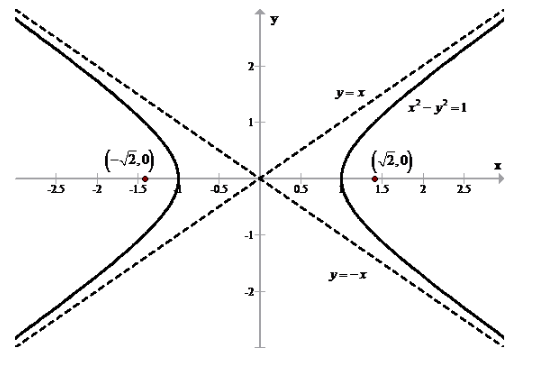# To find the foci and asymptotes of the hyperbola x 2 − y 2 = 1 and sketch its graph### Single Variable Calculus: Concepts...

4th Edition
James Stewart
Publisher: Cengage Learning
ISBN: 9781337687805### Single Variable Calculus: Concepts...

4th Edition
James Stewart
Publisher: Cengage Learning
ISBN: 9781337687805

#### Solutions

Chapter B, Problem 52E

(a)

To determine

## To calculate: To find the foci and asymptotes of the hyperbola x2−y2=1 and sketch its graph

Expert Solution

Foci of hyperbola are (2,0) and (2,0)

Asymptotes of hyperbola are y=±x

### Explanation of Solution

Given information: Equation of hyperbola is x2y2=1

Formula Used:

Standard equation of hyperbola is

x2a2y2b2=1 ,

where c2=a2+b2

Foci of above hyperbola is (±c,0)

Asymptotes of y=±bax

Calculation:

Equation of ellipse is given as

x2y2=1

Rewrite the equation as follows:

x212y212=1

On comparing the equation with standard equation of hyperbola,

a=1

b=1

Value of c is calculated as follows:

c2=a2+b2c2=12+12c2=1+1c2=2c=±2

Thus, foci of hyperbola are (2,0) and (2,0)

Now, asymptotes of hyperbola are calculated as

y=±11xy=±x

On sketching the hyperbola:Conclusion:

Hence, foci of hyperbola are (2,0) and (2,0) and asymptotes of hyperbola are y=±x

(b)

To determine

### To calculate: To sketch the graphof the hyperbola y2−x2=1

Expert Solution

The graph of hyperbola is sketched

### Explanation of Solution

Given information: Equation of hyperbola is x2y2=1

Formula Used:

Standard equation of hyperbola is

x2a2y2b2=1 ,

where c2=a2+b2

Foci of above hyperbola is (±c,0)

Asymptotes of y=±bax

Calculation:

Equation of ellipse is given as

y2x2=1

Foci and asymptotes are found out by reversing the roles of x and y

Thus, foci are (0,±c) and asymptotes are y=±bax

Using the values of equation x2y2=1

Thus, foci of hyperbola are (0,2) and (0,2)

Now, asymptotes of hyperbola are calculated as

y=±11xy=±x

On sketching the hyperbola:Conclusion:

Hence, graph of hyperbola is sketched

### Have a homework question?

Subscribe to bartleby learn! Ask subject matter experts 30 homework questions each month. Plus, you’ll have access to millions of step-by-step textbook answers!